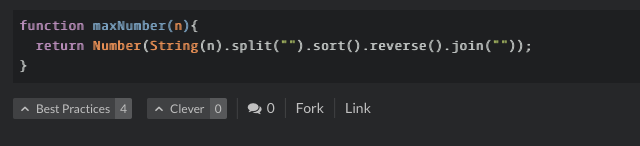# Reverse string questions

Greetings all !

So in my attempts to answer this question I found several methods of solving the process of reversing the string… all but one made sense to me… and I wanted to get some clarification, please .

``````function reverseString(str) {
if (str === "")
return "";
else
return reverseString(str.substr(1)) + str.charAt(0);
}
reverseString("hello");

``````

The question is/are I can see where the split occurs… in the “else” portion where it returns the string “elloh” after the first pass just a bit confused on how the string gets reversed… it appears to me that through each recursion it would simply end up just adding the next character to the end of the string ? like there almost needs to be another process ?

Thank you in advance for attempting to chisel into this grey matter !Your code has been blurred out to avoid spoiling a full working solution for other campers who may not yet want to see a complete solution. Please do not post full working solutions, but instead ask about specific lines of code and post only those lines.

Thank you.

try this:

``````let x = 1;
function factorial(n) {
if (n==1) return 1;                      // stops recursion / exits function
x *= n;                                  // updates x
console.log("x = " + x, " | n = " + n);  // logs values
return n * factorial(--n);               // multiplies previous n with new value (stacks)
}
``````

If you are having trouble understanding, it might be because your head is not wrapping around the idea of a function returning another function (in this case, itself).
If that is the case, then play around a bit with this idea and it will soon become clear how recursion works.

``````function test() {
return function nestedFunction() {
return "hello";
}
}
test()   // runs the function test() / returns the inside function

/* Since adding the first parenthesis returns a function,
** you can run the nestedFunction() by adding another
** set of parethensis
*/
test()() // returns "hello"
``````

With the strings it is about the same. It works kiiiiiind of like this:

``````function a(str = "hello") {
return (function a(str = "ello") {
return (function a(str = "llo") {
return (function a(str = "lo") {
return (function a(str = "o") {
return (function a(str = "") {
return "";
})()+"o";
})()+"l";
})()+"l";
})()+"e";
})()+"h";
}

a(); // logs "olleh"
``````

you can use

``````example
let a = "my name"
console.log(a.split(' ').reverse().join(' ')
``````
1 Likethis works and it is just a line. i would highly recommend analyzing challenge below. the challenge was:

1- take the numbers in the string, sort them and finally push them as a number.“375” => “3", “7", “5" => “3”,”5”,”7" => “7”,”5”,”3" => “753”=>753

this challenge from another place, helped me a lot. sort could be written better but since each number is < 10 => just sort() worked.